# Integration in Calculus Chapter Exam

Exam Instructions:

Choose your answers to the questions and click 'Next' to see the next set of questions. You can skip questions if you would like and come back to them later with the yellow "Go To First Skipped Question" button. When you have completed the practice exam, a green submit button will appear. Click it to see your results. Good luck!

### Page 1

#### Question 1 1. Evaluate the following integral. As a hint, you might want to simplify the fraction as shown.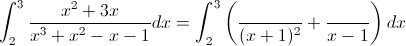#### Question 2 2. Evaluate the following integral.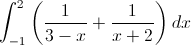#### Question 3 3. Simplify the following integral.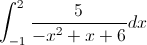#### Question 4 4. Evaluate.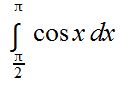### Page 2

#### Question 6 6. Which of the following is your end point?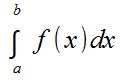#### Question 7 7. Which of the following is a possible solution when you integrate the expression below?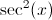#### Question 8 8. Integrate the following.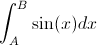#### Question 9 9. Integrate the following.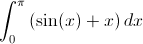#### Question 10 10. Evaluate the following.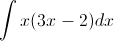### Page 3

#### Question 11 11. Calculate the integral.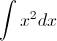#### Question 12 12. Assume the symbols are constants. Evaluate the following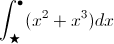#### Question 13 13. Which of the following is the BEST way to solve the integral below using integration by parts?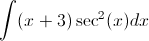#### Question 14 14. Evaluate the following.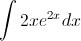#### Question 15 15. Use integration by parts to integrate the following: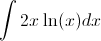### Page 4

#### Question 16 16. Evaluate the following integral.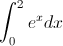#### Question 17 17. Evaluate the following integral.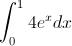#### Question 18 18. Evaluate the following integral.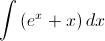#### Question 19 19. Which of the following integrals represents the area under the graph below?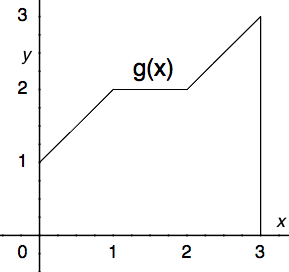#### Question 20 20. Given f(x) on the graph below, which of the following integrals will equal 0?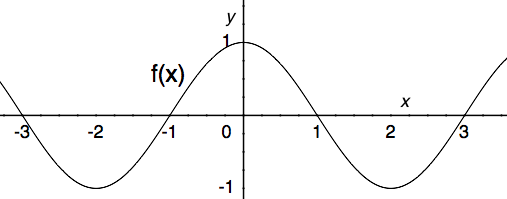### Page 5

#### Question 21 21. Which of the following graphs shows the correct region of integration for the integral below?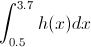#### Question 22 22. Evaluate the following integral.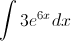#### Question 23 23. Evaluate the following integral.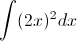#### Question 24 24. Evaluate the following integral.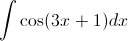#### Question 25 25. Evaluate the following integral.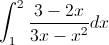### Page 6

#### Question 26 26. Evaluate.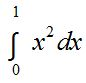#### Question 27 27. Integrate the following.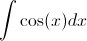#### Question 28 28. Evaluate the following integral.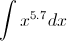#### Question 29 29. Use integration by part to integrate the following: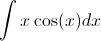#### Question 30 30. Evaluate the following integral.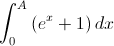#### Integration in Calculus Chapter Exam Instructions

Choose your answers to the questions and click 'Next' to see the next set of questions. You can skip questions if you would like and come back to them later with the yellow "Go To First Skipped Question" button. When you have completed the practice exam, a green submit button will appear. Click it to see your results. Good luck!

Support